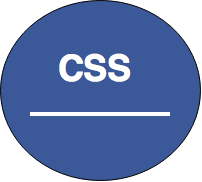# CSS Tables

Last Updated Jul 21, 2015, 12:00:06 PM

## CSS Tables

The table element in `HTML` is used for displaying tabular data. You can think of it as a way to describe and display data that would make sense in spreadsheet software. Essentially: columns and rows

Tables are created using the HTML elements, but we can style them using CSS. Every table can have a table heading, table rows, and columns. The data in tables are represented using rows and columns

HTML tables are created using the HTML <table> tag. You combine this tag with other tags that define each row and column within your table.

For example, take a look at the below table, this is a sample table that contains some rows and columns

Table cell 1 Table cell 2
Table cell 3 Table cell 4

## Table Borders

Table border is used to specify the border in `HTML` table. The property we use is `border`

The example below specifies a black border for <table>, <th>, and <td> elements:

Example
Output## Collapse Borders

The css `border-collapse` property is used to set whether the table borders are collapsed into a single border or seperated

The example HTML table would look like as follows

Let's take a look at how the css would look like for the above HTML table

The above example would apply the css`border-collapse` to the table and set its border as red with 3px

Output## Table Width and Height

CSS `width` and `height` property is used to define the height and width of a table

The example below sets the `width` of the table to 80%, and the `height` of the <th> elements to 40px:

The example HTML table would look like this:

Example

Let's look at how the css code would look like for the above table using the css`width and height` property

Output## Horizontal Text Alignment

If you notice, that the table headers are by default set to left. However using the css`text-align` property we can set the table headers to left, right or center.
Example

The following example right-aligns the text in <th> elements:

The example HTML table would look like

Now let's look at how the css code would look like for the above table using css`text-align` property

Output

If the css property is set to `text-align:right` then the output would look like below

Notice the table headersIf the css property is set to `text-align:left` then the output would look like belowIf the css property is set to `text-align:center` then the output would look like below## Vertical Text Alignment

The `vertical-align` property sets the vertical alignment, like top, bottom, or middle.

The following example sets the vertical text alignment to bottom for <td> elements:

The example html table would look like below:

### Example

Let's look at how the css code would look like after applying the css`vertical-align ` property

Output## Table Color

CSS `table-color` property is used to set the table color
Example

Let's look at the css code for the above table with css table `color` property

OutputSources and Credits

The source of the content has been referred and updated with Mozilla Foundation and W3C Organization

Last Updated Jul 21, 2015, 12:00:06 PM